# Filter Transforms

#### 2020-04-20

In astronomy we often have filters that are similar but annoyingly different to each other. For instance the Sloan $$ugri$$ filter set are all similar to, but not identical to, the VST $$ugri$$ filters. In fact in detail probably almost no two telescopes have identical versions of the same filters (even if their names imply that they do). But no fear, ProSpect can help fix this with relative ease!

In general to solve this problem you will need two nearby filters in one system to the one you want to fix in another, e.g. Sloan $$r_S$$ and $$i_S$$ can be used to convert the Sloan $$r_S$$ to VST $$r_V$$.

## Setting Things Up

Load the libraries we will need:

library(ProSpect)
library(hyper.fit)
## Loading required package: magicaxis
## Loading required package: MASS
## Loading required package: rgl
## Loading required package: LaplacesDemon
##
## Attaching package: 'LaplacesDemon'
## The following objects are masked from 'package:pracma':
##
##     logit, loglog, Mode
library(data.table)
library(foreach)
library(magicaxis)

Load some data we want to use:

data("BC03lr") #BC03 spectral library
data("Dale_NormTot") #Normalised Dale templates

Preprocess filters:

filters =c('r_SDSS','i_SDSS','r_VST')
filtout = foreach(i = filters)%do%{approxfun(getfilt(i))}
names(filtout) = filters

We are interested in comparing the Sloan and VST $$r$$ bands so let’s see what these look like:

magcurve(filtout[](x), 5000, 7500, ylim=c(0,1), grid=TRUE, xlab='Wave / Ang', ylab='Norm')
legend('topleft', legend=c('r_SDSS','r_VST'), col=c('black','red'), lty=1)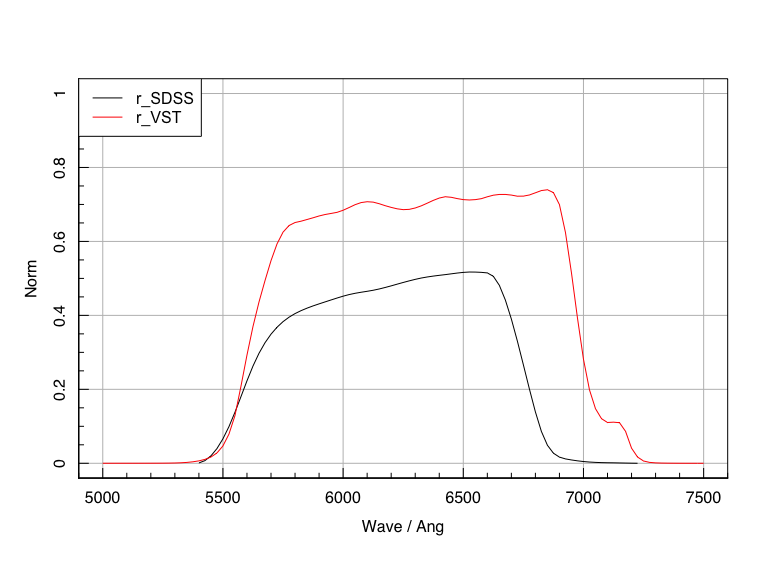The normalisation of filter throughput does not matter (so ignore the vertical offset), but what is clear is that the VST filter stretches bluewards compared to SDSS. This is significant since steep spectral shapes at low redshift in this regime means the VST $$r$$ band will usually be a bit brighter than SDSS.

## Predicting Filters

Make some random SFHs with redshifts over the range of interest. The first 5 arguments here control the SFH, and the last is a Uniform sampling of the redshift. This last part might need to be adapted to better match extreme samples (very high redshift), where an additional use of the redshift dependency might improve the converstion.

set.seed(666)

params = cbind(runif(1e3), runif(1e3), runif(1e3), runif(1e3), runif(1e3), runif(1e3,0,0.5))

ranSFHs = foreach(i = 1:1e3, .combine='rbind')%do%{
ProSpectSED(m1=params[i,1], m2=params[i,2], m3=params[i,3], m4=params[i,4], m5=params[i,5],
z=params[i,6], filtout=filtout, speclib=BC03lr, Dale=Dale_NormTot,
returnall = FALSE)
}
ranSFHs = data.table(ranSFHs)
colnames(ranSFHs)=filters
ranSFHs[,mag_rs:=Jansky2magAB(r_SDSS)]
ranSFHs[,mag_is:=Jansky2magAB(i_SDSS)]
ranSFHs[,mag_rv:=Jansky2magAB(r_VST)]
ranSFHs[,rs_rv:=mag_rs-mag_rv] # to make r_SDSS minus r)VST colours
ranSFHs[,rs_is:=mag_rs-mag_is] # to make r_SDSS minus i_SDSS colours

We can see how close the Sloan and VST $$r$$ bands really are:

magplot(density(ranSFHs[,mag_rv-mag_rs]), grid=TRUE, xlab='rv - ri')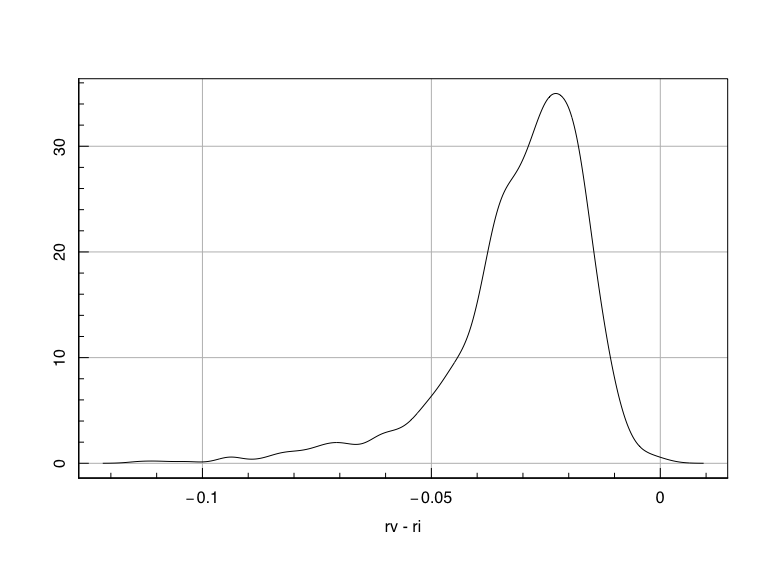It is clear there is a strong shift of around 0.03 mag, with the VST filter appearing to be brighter because it is a bit redder, and template shapes at these redshifts mean a bit redder is a bit brighter.

fitout = hyper.fit(ranSFHs[,list(rs_is, rs_rv)])

Plot it:

plot(fitout, grid=TRUE)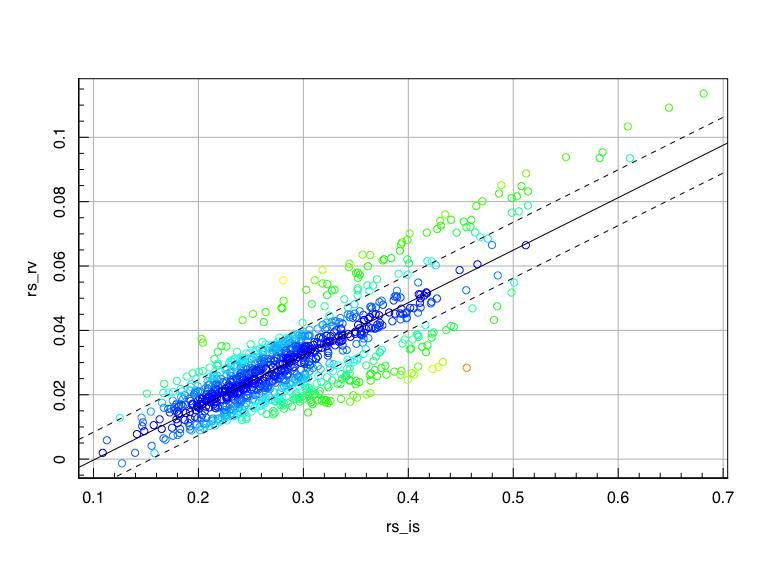Check the output:

summary(fitout)
## Call used was:
##
## hyper.fit(X = ranSFHs[, list(rs_is, rs_rv)])
##
## Data supplied was 1000rows x 2cols.
##
## Requested parameters:
##
##       alpha1    beta.vert    scat.vert
##  0.163072562 -0.016604277  0.008663928
##
## Errors for parameters:
##
##    err_alpha1 err_beta.vert err_scat.vert
##  0.0035707333  0.0010667827  0.0001823016
##
## The full parameter covariance matrix:
##
##                  alpha1     beta.vert     scat.vert
## alpha1     1.275014e-05 -3.681427e-06  1.605182e-08
## beta.vert -3.681427e-06  1.138025e-06 -4.638632e-09
## scat.vert  1.605182e-08 -4.638632e-09  3.323387e-08
##
## The sum of the log-likelihood for the best fit parameters:
##
##       LL
## 4261.728
##
## Unbiased population estimator for the intrinsic scatter:
##
##   scat.vert
## 0.008672605
##
## Standardised parameters vertically projected along dimension 2 (rs_rv):
##
##       alpha1    beta.vert    scat.vert
##  0.163072562 -0.016604277  0.008663928
##
## Standardised generative hyperplane equation with unbiased population
## estimator for the intrinsic scatter, vertically projected along dimension 2 (rs_rv):
##
## rs_rv ~ N(mu= 0.1631*rs_is - 0.0166 , sigma= 0.008673)

With a bit of re-arrangement we get to:

$r_v = r_s - 0.1723 (r_s - i_s) + 0.0173$ Let’s check that this works well:

 ranSFHs[,mag_rv_pred:=mag_rs - 0.1723*(rs_is)+0.0173]

And now we can see if we have fixed things:

magplot(density(ranSFHs[,mag_rv-mag_rs]), grid=TRUE, xlab='r - r', xlim=c(-0.2,0.2),
ylim=c(0,50))
lines(density(ranSFHs[,mag_rv-ranSFHs$mag_rv_pred]), col='red') legend('topleft', legend=c('rv - rs', 'rv - rv_pred'), col=c('black', 'red'), lty=1)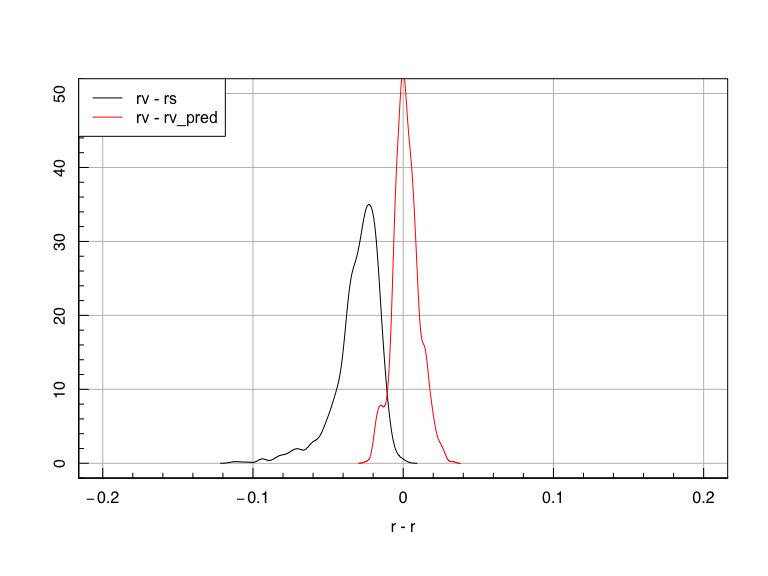Da dah! ## Now We Heave Learnt What To Do… ProSpect offers a very high level routine to do all of this in one magical go. Here we will try it using three different input filters to best map the VST $$r$$ band: filters =c('g_SDSS','r_SDSS','i_SDSS','r_VST') filt_in = foreach(i = filters[1:3])%do%{approxfun(getfilt(i))} filt_out = approxfun(getfilt(filters)) names(filt_in) = filters[1:3] #vital we give our input filters useful names This can all be run through the above steps in all adjacent band combinations with: trans = filterTranBands(filt_in=filt_in, filt_out=filt_out) print(trans) ##$g_SDSS + alpha.(g_SDSS - r_SDSS) + beta +/- sigma
##       alpha        beta       sigma
## -1.02361081 -0.01163874  0.01375764
##
## $r_SDSS + alpha.(g_SDSS - r_SDSS) + beta +/- sigma ## alpha beta sigma ## -0.02361081 -0.01163874 0.01375764 ## ##$r_SDSS + alpha.(r_SDSS - i_SDSS) + beta +/- sigma
##        alpha         beta        sigma
## -0.161059313  0.016022485  0.008666708
##
## $i_SDSS + alpha.(r_SDSS - i_SDSS) + beta +/- sigma ## alpha beta sigma ## 0.838940687 0.016022485 0.008666708 In general we want the filter transform that minimises the vertical scatter (sigma above). This happens to be the transform we computed manually using the SDSS $$r$$ and $$i$$ bands. ## Using your own filters If you have your own filter file where the columns are wavelength (in Angstroms, named ‘wave’) and throughput (in photons not energy, named ‘response’) then it is fairly simple to compute your own filter conversions based on the following example. Note the throughput/response does not need to be normalised to anything in particular (so does not need to integrate to 1.0, or have a max value of 1.0 etc)- this is done internally. First we need to create some fake filter file data. For simplicity we will just put this in a random temp file: filt_temp_r = tempfile() write.table(getfilt('r_SDSS'), file=filt_temp_r) For most users, the below is where you enter the problem- you already have some filter data in the correct format and you wish to read this in: filt_r = read.table(filt_temp_r) filt_r[1:5,] ## wave response ## 1 5380 0.0000 ## 2 5405 0.0012 ## 3 5430 0.0087 ## 4 5455 0.0228 ## 5 5480 0.0440 We can check this looks okay: magplot(filt_r, type='l', xlab='Wave / Ang', ylab='Norm', grid=TRUE)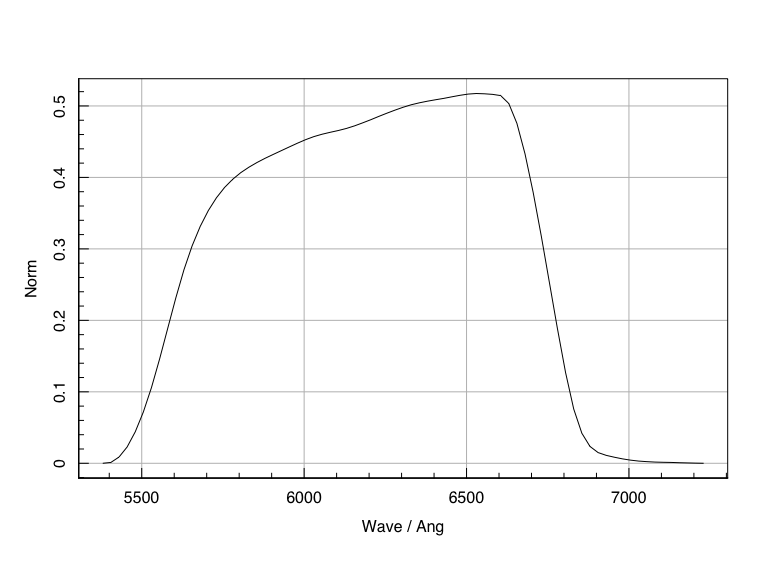We will now do a similar thing with our g and i data: filt_temp_g = tempfile() write.table(getfilt('g_SDSS'), file=filt_temp_g) filt_g = read.table(filt_temp_g) filt_temp_i = tempfile() write.table(getfilt('i_SDSS'), file=filt_temp_i) filt_i = read.table(filt_temp_i) We now need to put this into the list structure that filterTranBands expects. First we define out input filter set that we wish to transform from (we can have lots of these): filt_in = list( g_SDSS = filt_g, # note faster if wrapped in approxfun(filt_X) etc r_SDSS = filt_r, i_SDSS = filt_i ) Now we define our target filter that we want to transform to (we can have just one of these): filt_out = getfilt('r_VST') # note faster if wrapped in approxfun(filt_X) etc And we can check that this long-winded (but informative for those loading in their own filters) way round gives the same answers as before: trans = filterTranBands(filt_in=filt_in, filt_out=filt_out) print(trans) ##$g_SDSS + alpha.(g_SDSS - r_SDSS) + beta +/- sigma
##       alpha        beta       sigma
## -1.02361081 -0.01163874  0.01375764
##
## $r_SDSS + alpha.(g_SDSS - r_SDSS) + beta +/- sigma ## alpha beta sigma ## -0.02361081 -0.01163874 0.01375764 ## ##$r_SDSS + alpha.(r_SDSS - i_SDSS) + beta +/- sigma
##        alpha         beta        sigma
## -0.161059313  0.016022485  0.008666708
##
## \$i_SDSS + alpha.(r_SDSS - i_SDSS) + beta +/- sigma
##       alpha        beta       sigma
## 0.838940687 0.016022485 0.008666708

Yes they do!